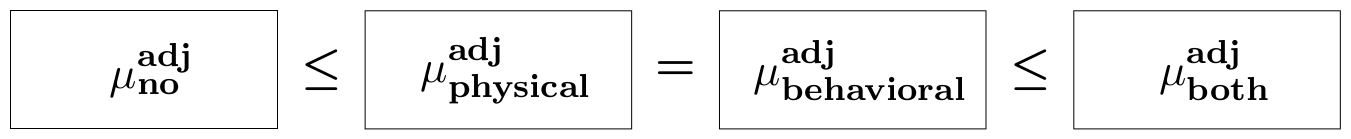#### Ordered adjusted means in an ANCOVA layout

In this example we consider the AngerManagement dataset, which is built-in restriktor. The data consists of a persons' decrease in aggression level between week 1 (intake) and week 8 (end of training) for four different treatment groups of anger management training, namely (1) no training, (2) physical training, (3) behavioral therapy, and (4) a combination of physical exercise and behavioral therapy. In addition, the dataset includes the covariate age. The purpose of the study was to test the assumption that the exercises would be associated with a reduction in the mean aggression levels with respect to the covariate age. In particular, the hypothesis of interest is:

$$H^{adj}_1: \mu^{adj}_{No} \leq \big[\mu^{adj}_{Physical} = \mu^{adj}_{behavioral}\big] \leq \mu^{adj}_{Both},$$

where $\mu^{adj}_j$ denotes the population adjusted mean for group $j$. This hypothesis states that the decrease in aggression levels is smallest for the 'no training' group, larger for the 'physical training' group and “behavioral therapy' group, with no preference for either method, and largest in the 'combination of physical exercise and behavioral therapy' group. Below a graphical representation of the order-constrained hypothesis.In what follows, we describe all steps to test this hypothesis.

### Step 1. - load the restriktor library

library(restriktor)


### Step 2. - import data

The AngerManagement dataset is available in restriktor and can be called directly, see next step.

### Step 3. - center the covariates

To obtain adjusted means, the easiest way is by creating a new variable where the covariate 'age' is centered at its average. This can be done as follows: